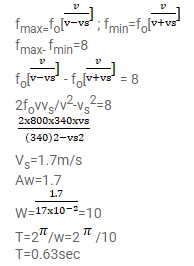# A small source of sound oscillates in simple harmonic motion withQuestion:

A small source of sound oscillates in simple harmonic motion with an amplitude of $17 \mathrm{~cm}$. A detector is placed along the line of motion of the source. The source emits a sound of frequency $800 \mathrm{~Hz}$ which travels at a speed of $340 \mathrm{~m} \mathrm{~s}^{-1}$. If the width of the frequency band detected by the detector is $8 \mathrm{~Hz}$, find the time period of the source.

Solution: